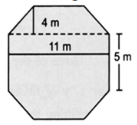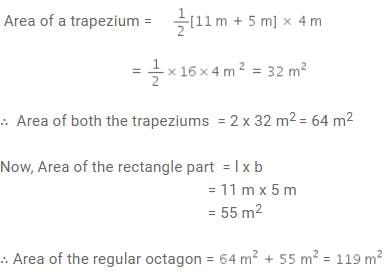# Test: Mensuration- 2

## 10 Questions MCQ Test IBPS PO Prelims- Study Material, Online Tests, Previous Year | Test: Mensuration- 2

Description
Attempt Test: Mensuration- 2 | 10 questions in 10 minutes | Mock test for Class 8 preparation | Free important questions MCQ to study IBPS PO Prelims- Study Material, Online Tests, Previous Year for Class 8 Exam | Download free PDF with solutions
QUESTION: 1

### Find the volume of a cuboid whose length is 8 cm, breadth 6 cm and height 3.5 cm.

Solution: (8×6)×3.5=48×3.5= 168.00cm cube.
QUESTION: 2

### Find the volume of a cuboid whose length is 8 cm, width is 3 cm and height is 5 cm.

Solution: Volume of cubiod =l×b×h
=8×3×5
=120cm3 .
QUESTION: 3

### Find the perimeter of the a square with side 4cm.

Solution: Because the perimeter of square is 4×side
so, 4×4=16
QUESTION: 4

Find the cube root of 140 × 2450.

Solution:

140 x 2450 = 343000
Cube root of 343000 = (343000)1/3 = (703)1/3 = 70

QUESTION: 5

A rectangular paper of width 7 cm is rolled along its width and a cylinder of radius 20 cm is formed. Find the volume of the cylinder.

Solution:
Given  : A rectangular paper of width 14 cm is rolled along its width to form a cylinder.

Height of cylinder =  h  =  7 cm = width of the rectangular paper

And
Radius of cylinder ( Given ) =  r  = 20 cm

And
we know Volume of cylinder  = π r^2 h , So

Volume of our given cylinder = 22/7 x 20 x 20 x 7  = 8800 cm^3
QUESTION: 6

Top surface of a raised platform is in the shape of regular octagon as shown in the figure. Find the area of the octagonal surface.Solution:

This regular octagon can be into two trapeziums (each having height 4 m and parallel sides as 11 m and 5 m) and a rectangular part with length 11 m and breadth 5 m.​QUESTION: 7

The diagonal of a quadrilateral shaped field is 24 m and perpendicular dropped on it from the remaining opposite vertices are 6 m and 12 m. Find the area of the field.

Solution:

area=1/2×d×(h1+h2)
=1/2×24×(12+6)
=12(18)
=216 m2

QUESTION: 8

If the parallel sides of a parallelogram are 2 cm apart and their sum is 10 cm then its area is:

Solution:
QUESTION: 9

If the edge of a cube is 1 cm then which of the following is its total surface area?

Solution: Total surface area of cube is 6*length^2
=6×1^2
=6×1
=6 cm2
QUESTION: 10

Find the area of a triangle whose base is 4 cm and altitude is 6 cm.

Solution:

We know that area of triangle is equals to 1/2 base × altitude.
Here, base = 4 cm and altitude = 6 cm.
So, area = 1/2 × 4 × 6= 24 /2= 12 cm2.Use Code STAYHOME200 and get INR 200 additional OFF Use Coupon Code